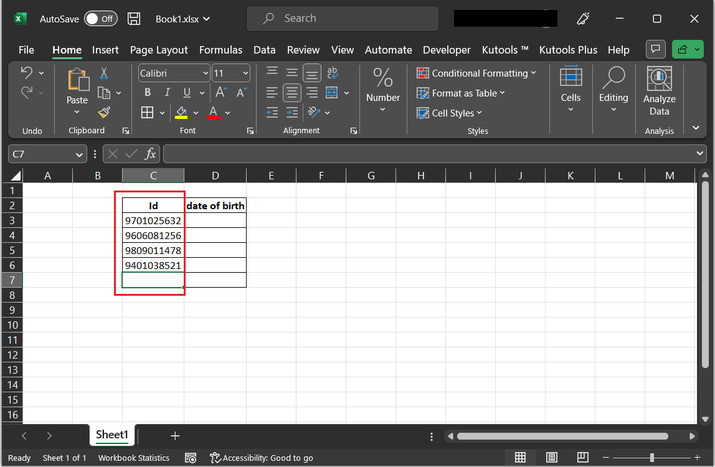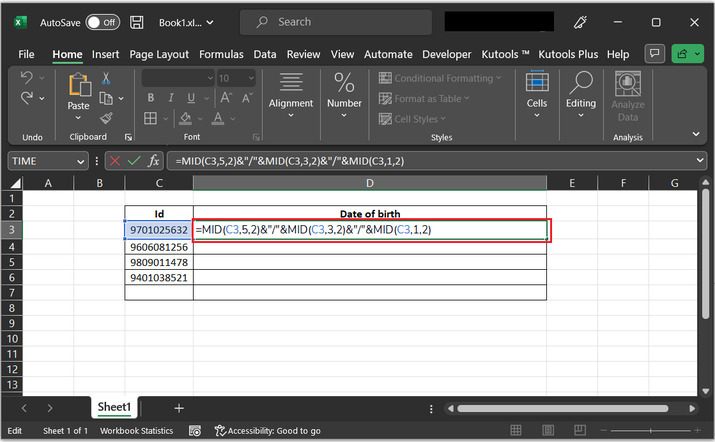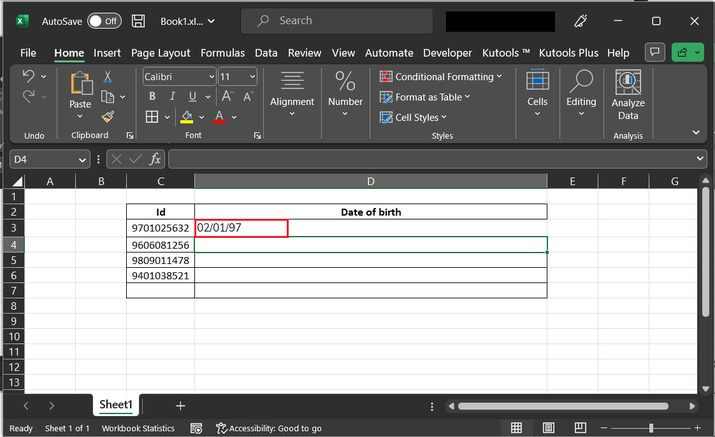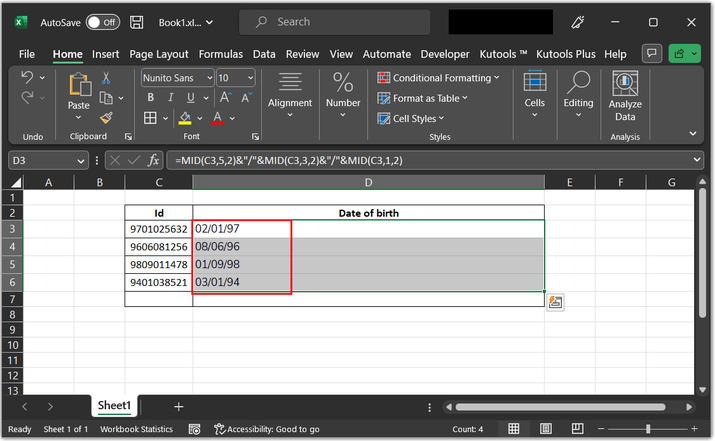# How to get date of birth from ID number in Excel?

In this article the user will understand the process of generating the date of birth from the ID number in excel. This article provides a brief and an easy example to guide the user the way to generate the date of birth from the provided employee ID. Thus, to evaluate the date of birth the user needs to process the provided data in such a way that the date of birth will be evaluated effectively without any miss. The provided example uses the formula to calculate the date of birth from the provided ID number. Here, will be assuming that the ID column, is a number without any decimal place.

## Example 1: To generate date of birth from ID number in excel by using the user defined formula

Step 1

Consider the given worksheet below with two columns. The first column contains ID number. The first two digits of the Id column represent the birth year, the third and fourth pair of digits represent the birthday, and month. In the example, user will understand the process to evaluate date of birth for such provide id.

To understand more precisely, consider the number “9701025632”. Here, 97 is the year of birth, 01 is the month of birth, and 02 is the date of birth. Rest remaining portion of the id cannot have any relation with date of birth.Step 2

Go to the D2, cell and paste the provided formula “=MID(C3,5,2)&"/"&MID(C3,3,2)&"/"&MID(C3,1,2)”. In this formula, the user will evaluate birth year, month and date, and will add the processed data along with a “/” symbol, to represent date in proper format. Consider below provided image for reference:Explanation for above provided formula

The provided excel formula converts a number into a date. In the provided formula, will use a MID function to extract the data such as day, month, and year components to create a date value in the desired format.

Stepwise explanation

• MID(C3,5,2) - This part of formula, use the MID function to extract the day component from the date available in the C3 cell. The first argument, C3, specifies the cell containing the date. The second argument, 5, shows the position of the day component within the date string (counting from the left). The third argument, 2, shows the length of the day component.

• &"/"& - The provided symbol concatenates the day component with a forward slash ("/").

• MID(C3,3,2) - This part uses the MID function to extract the month component from the date provided in the C3 cell. The first argument, C3, shows that the cell contains the date. The second argument, 3, shows the position of the month component within the date string (counting from the left). The third argument, 2, shows the length of the month component.

• &"/"& - This concatenates the month component with another forward slash ("/").

• MID(C3,1,2) - This expression uses the MID function to extract the year component from the date in cell C3. The first argument, C3, specifies the cell containing the date. The second argument, 1, represents the position of the year component within the date string (counting from the left). The third argument, 2, represents the length of the year component.

The result is a text string that combines the day, month, and year components of the date in cell C3, separated by forward slashes.

Step 3

After typing the above formula, press “Enter” key. This will display the result to the D3 cell automatically.Step 4

Click on the D3 cell and drag the plus “+” sign to the bottom of the 6 th row. This will display the results, as shown below:## Conclusion

In this article user will be able to understand the process to evaluate the data with the help of provided stepwise explanation. Every step contains a relevant snapshot for data. By referring all the steps clearly every user can evaluate the age from ID.

Updated on: 28-Aug-2023

1K+ Views